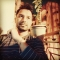## C++ Mathematical Functionssudhir sharma

Updated on 19-Sep-2019 07:05:50

Mathematical calculations can be done in C++ programming language using the mathematical functions which are included in math or cmath library. These mathematical functions are defined to do complex mathematical calculations. Let’s learn each of them one by one −sineThe sin method is used to calculate the sin of the ... Read More

## C++ map having key as a user defined data typesudhir sharma

Updated on 19-Sep-2019 06:52:55

A map is a data structure that stores information in the form of key and value pairs. In C++, map is defined in STL (standard template library) and store keys in an ordered form.Syntax to define a map −map map_name;The data type of any of these two data of the ... Read More

## C++ interview questions on virtual function and abstract classsudhir sharma

Updated on 19-Sep-2019 06:44:52

What is a virtual function?A virtual function is a method that does not have a definition when defined in the base class. This method is left black in the parent class and it is redefined in the child class.What is an abstract class?An abstract class is a class that has ... Read More

## C Program for Rat in a Maze - Backtracking-2?sudhir sharma

Updated on 20-Aug-2019 10:25:14

Rat in a maze is also one popular problem that utilizes backtracking. IA maze is a 2D matrix in which some cells are blocked. One of the cells is the source cell, from where we have to start. And another one of them is the destination, where we have to ... Read More

## C++ Program to remove spaces from a string?sudhir sharma

Updated on 20-Aug-2019 09:02:02

The program takes a string and removes the spaces in it. This is useful when we want to save the space of our The following sample shows how it is done with an explanation.Input: Hello World Output: HelloWorldExplanationTo remove or delete spaces from the string or sentence, you have to ... Read More

## C/C++ Program for Linear Search?sudhir sharma

Updated on 20-Aug-2019 09:00:05

In linear search algorithm, we compare targeted element with each element of the array. If the element is found then its position is displayed.The worst case time complexity for linear search is O(n).Input: arr[] = { 12, 35, 69, 74, 165, 54} Sea=165 Output: 165 is present at location 5.Explanationlinear ... Read More

## C++ Program to find whether a number is the power of two?sudhir sharma

Updated on 20-Aug-2019 08:57:26

Check if a given number is a power of 2. First check below which numbers are the power of two or not. This code checks whether the number is odd and then divide it concurrently until it becomes 0 or odd. If it becomes 0 then it is a power ... Read More

## C++ Program for cube sum of first n natural numbers?sudhir sharma

Updated on 20-Aug-2019 08:55:36

Positive integers 1, 2, 3, 4... are known as natural numbers.This program takes a positive integer from the user ( suppose user-entered n ) then, this program displays the value of 13+23+33+....+n3.Input: n = 3 Output: 36Explanation13+23+33 = 1 +8+27 = 36This program takes a positive integer from user( suppose ... Read More

## C++ Program for Cycle Sort?sudhir sharma

Updated on 20-Aug-2019 08:54:14

Cycle sort is an in-place, unstable sorting algorithm, a comparison sort that is theoretically optimal in terms of the total number of writes to the original array, unlike any other in-place sorting algorithm. It is based on the idea that the permutation to be sorted can be factored into cycles, ... Read More

## C Program to Find the minimum sum of factors of a number?sudhir sharma

Updated on 20-Aug-2019 08:50:59

The program to find the minimum sum of factors of a number. The logic for solving this problem is, find all the set of factors and adding them. For every set of factors, we will do the same and then compare all of them. Then find all the minimum of ... Read More